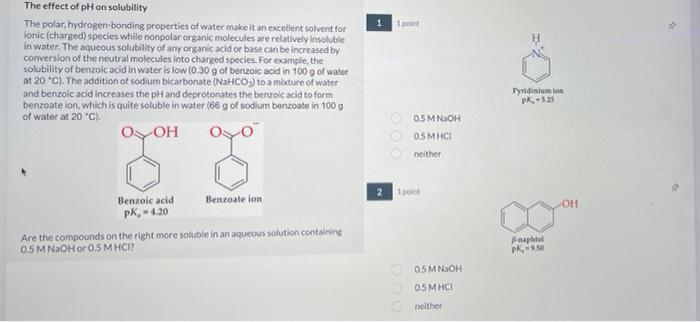Home / Expert Answers / Chemistry / the-effect-of-mathrm-ph-on-solubility-the-polar-hydrogen-bonding-properties-of-water-make-pa818

# (Solved): The effect of $$\mathrm{pH}$$ on solubility The polar, hydrogen-bonding properties of water make ...The effect of $$\mathrm{pH}$$ on solubility The polar, hydrogen-bonding properties of water make it an excellent solvent for ionic (charged) species while nonpolar organic molecules are relatively insoluble in water. The aqueous solubility of ary organic acid or base can be increased by conversion of the neutral molecules into charged species. For example, the solubility of benzolc acid in water is low $$(0.30 \mathrm{~g}$$ of benzoic acid in $$100 \mathrm{~g}$$ of water at $$20^{\circ} \mathrm{C}$$ ). The addition of sodium bicarbonate (NaHCO ) to a mbeture of water and benzolc acid increases the pH and deprotonates the benzolc acid to form benzoate ion, which is quite soluble in water $$(66 \mathrm{~g}$$ of sodium benzoate in $$100 \mathrm{~g}$$ of water at $$20^{\circ} \mathrm{Cl}$$. 1 point The components of poison ivy and poison oak that produce itchy rash are catechols substituted with long-chain alkyl groups:

We have an Answer from Expert